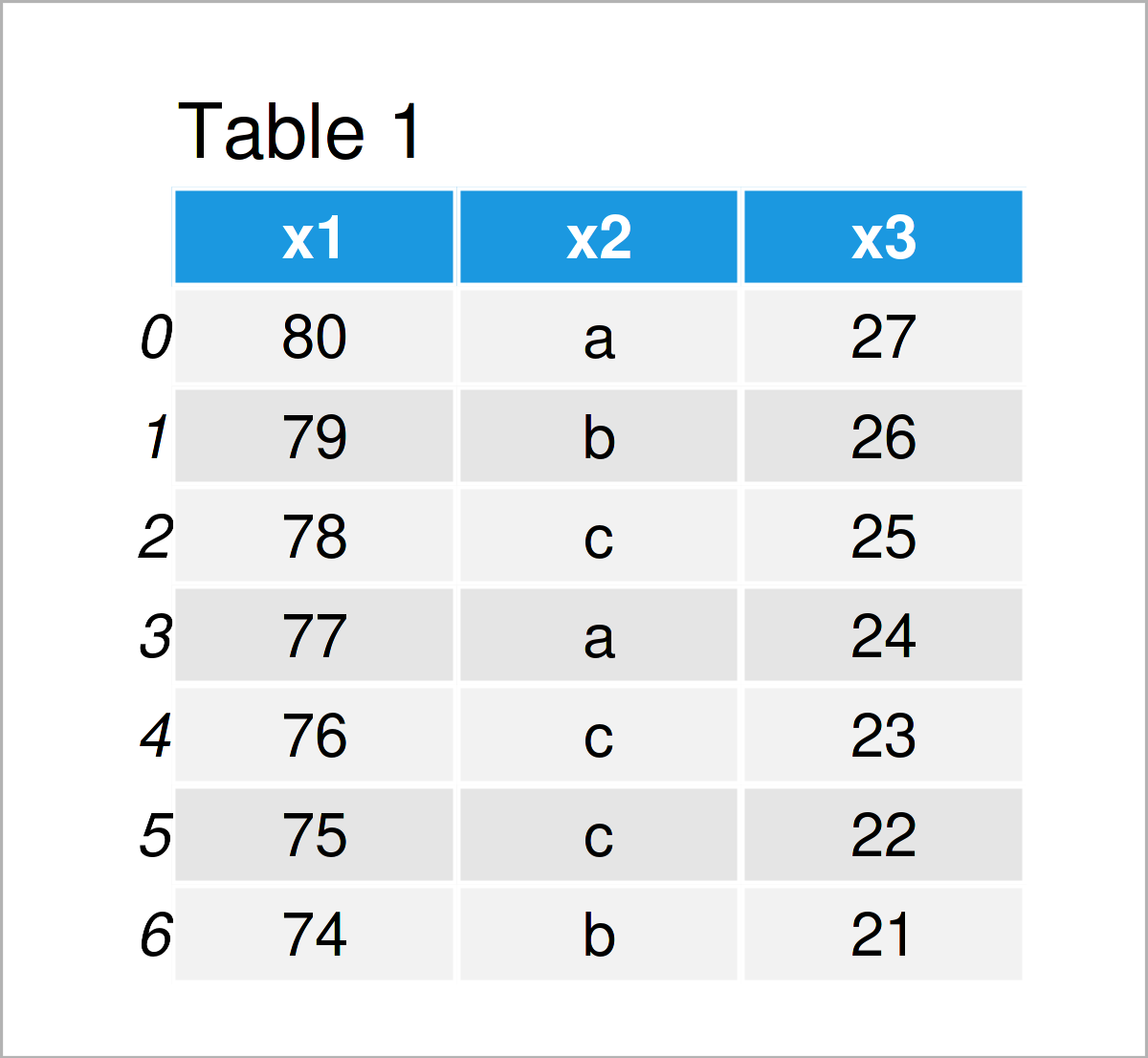# Search Value in pandas DataFrame in Python (2 Examples)

In this tutorial you’ll learn how to locate a specific value in a pandas DataFrame in the Python programming language.

The article looks as follows:

Let’s dive into it.

## Example Data & Add-On Libraries

In order to use the functions of the pandas library, we first have to import pandas:

`import pandas as pd                              # Import pandas library in Python`

As a next step, I also need to create some example data:

```data = pd.DataFrame({'x1':range(80, 73, - 1),    # Create pandas DataFrame
'x2':['a', 'b', 'c', 'a', 'c', 'c', 'b'],
'x3':range(27, 20, - 1)})
print(data)                                      # Print pandas DataFrame```Table 1 shows that our pandas DataFrame consists of seven lines and three columns.

## Example 1: Return Matrix of Logicals Indicating Location of Particular Value

In Example 1, I’ll illustrate how to create and print a data matrix containing logical values that indicate whether a data cell contains a particular value.

Let’s assume that we want to find out which elements in our example DataFrame contain the character ‘b’. Then, we can apply the isin function as shown below:

```search_result_1 = data.isin(['b'])               # Create matrix of logical values
print(search_result_1)                           # Print output```By executing the previous Python syntax, we have constructed Table 2, i.e. a matrix of logicals that indicates the locations of the element ‘b’ in our input data set.

## Example 2: Test if Value is Contained in pandas DataFrame Column

In this example, I’ll illustrate how to check if an entire column contains a certain value at least once.

To achieve this, we have to use the any function in addition to the isin function as shown below:

```search_result_2 = data.isin(['b']).any()         # Check by column
print(search_result_2)                           # Print output
# x1    False
# x2     True
# x3    False
# dtype: bool```

The previous output shows that only the second variable x2 contains the character ‘b’.

## Video, Further Resources & Summary

I have recently released a video on my YouTube channel, which illustrates how to search and find particular values in a pandas DataFrame. You can find the video below.

Please accept YouTube cookies to play this video. By accepting you will be accessing content from YouTube, a service provided by an external third party.If you accept this notice, your choice will be saved and the page will refresh.

Furthermore, you may want to have a look at the other articles on this website.

In this tutorial, I have shown how to search, find, and locate a specific value in a pandas DataFrame in the Python programming language. In case you have additional questions and/or comments, don’t hesitate to let me know in the comments section.

Subscribe to the Statistics Globe Newsletter